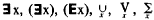# quantifier

(redirected from Quantifiers)
Also found in: Dictionary, Thesaurus.

## quantifier

[′kwän·tə‚fī·ər]
(mathematics)
Either of the phrases “for all” and “there exists”; these are symbolized respectively by an inverted A and a backward E.

## Quantifier

(from the Latin quantum, “how much”), a logical operation that gives the quantitative character of the range of objects with which the expression obtained as a result of its application is concerned. In ordinary language, words of the type “all,” “each,” “some,” “there exists,” “there is,” “any,” “every,” “unique,” “several,” “infinitely many,” “a finite number,” as well as all cardinal numbers, serve as conveyors of these characteristics. In formal languages in which predicate calculus is a constituent part, two kinds of quantifier turn out to be sufficient for the expression of all such characteristics: the universal quantifier (“for all x,” denoted by ∀x, (∀x), (x), (Ax),) and the existential quantifier (“for some x,” denoted by,). With the aid of quantifiers it is possible to write down the four fundamental forms of judgment of traditional logic: “all A are B” is written as ∀x [A(x) ⊃ B(x)], “no A is B” as ∀x[A(x)⊃ ∼ B(x)], “some A are B” as ∃ x [A (x) & B(x)], and “some A are not B” as ∃x[A(x)]; here A(x) denotes that x possesses the property A, ⊃ is the implication sign, ∼ is the negation sign, and & is the conjunction sign.

The part of a formula over which the operation of any quantifier is distributed is called the scope of operation of that quantifier (it may be indicated by parentheses). The entry of any variable into the formula directly after the quantifier or within the scope of the quantifier after which the variable stands is called its bound entry. All remaining variable entries are called free. A formula containing free variable entries is dependent on them (is a function of them), but the bound entries may be “renamed”; for example, the expressions ∃x (x = 2y) and ∃z(z = 2y) denote one and the same thing, but the same cannot be said of ∃x(x =2y) and ∃x(x = 2t). The use of quantifiers reduces the number of free variables in logical expressions and, if the quantifier is not a “dummy” (that is, if it is related to a variable actually entering into the formula), it transforms a three-place predicate into a two-place one, a two-place one into a single-place one, and single-place one into a proposition. The use of quantifiers is codified by special “quantification postulates” (whose addition to propositional calculus essentially implies an expansion of the latter into predicate calculus)—for example, the “Bernays’ postulates”: the axioms A(t)⊃ ∃xA(x) and ∀xA(x) ∃ A(t) and the rules of deduction “if it has been proved that CA(x), then it may be considered also proved that CxA(x)” and “if it has been proved that A(x)⊃ C” then it may be considered also proved that ∃xA(x)⊃ C” (here x does not enter freely into C).

Other kinds of quantifiers may be reduced to the universal and existential quantifiers. For example, in place of the uniqueness quantifier ∃!x (“there exists a unique x such that”) it is possible to write “ordinary” quantifiers, replacing ∃!xA(x) with

xA(x) & ∀yz [A(y) &A(z) ⊃ y = z]

Analogously, quantifiers “bound” to a single-place predicate P(x) —∃xpx) (“there exists an x satisfying the property P and such that”) and ∀x(p)x (“for all x satisfying the property P it is true that”)—can easily be expressed by means of the universal and existential quantifiers and the implication and conjunction operators:

xP(x)A(x) ≡ ∃x[P(x) & A(x)]]

and

xP(x)A(x) ≡ ∀x[P(x)A(x)]

### REFERENCES

Kleene, S. C. Vvedenie v metamatematiku. Moscow, 1957. Pages 72–80, 130–38. (Translated from English.)
Church, A. Vvedenie v matematicheskuiu logiku, vol. 1. Moscow, 1960. Pages 42–48. (Translated from English.)

IU. A. GASTEV

## quantifier

(logic)
An operator in predicate logic specifying for which values of a variable a formula is true. Universally quantified means "for all values" (written with an inverted A, LaTeX \forall) and existentially quantified means "there exists some value" (written with a reversed E, LaTeX \exists). To be unambiguous, the set to which the values of the variable belong should be specified, though this is often omitted when it is clear from the context (the "universe of discourse"). E.g.

Forall x . P(x) <=> not (Exists x . not P(x))

meaning that any x (in some unspecified set) has property P which is equivalent to saying that there does not exist any x which does not have the property.

If a variable is not quantified then it is a free variable. In logic programming this usually means that it is actually universally quantified.